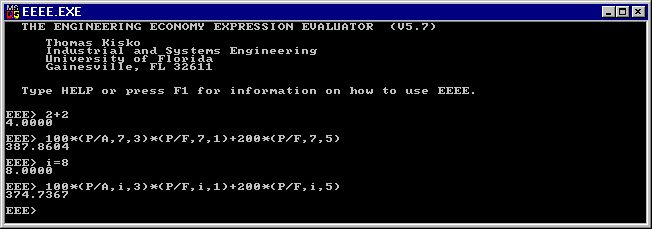THE ENGINEERING ECONOMY EXPRESSION EVALUATOR

Freeware by Thomas Kisko, P.E.
Industrial and Systems Engineering Department
University of Florida
303 Weil Hall
Gainesville, Florida 32611

Students and practitioners of engineering economy are faced with the unenviable task of evaluating engineering economy expressions. If the example expression:
1000 * (P/F,10,5) - 500 * (P/F,6,20) + 100 * (P/A,8,20)
were solved manually, it would require three table look-ups to determine the values of the cash flow factors. Even with a spreadsheet, determining the value of the expression would take some work.

The Engineering Economy Expression Evaluator, EEEE, is a public domain, PC compatible, computer program that solves engineering economy expressions. The above expression can be entered into EEEE exactly as written, and the computer will respond with the evaluated result.

Other features of EEEE include: plotting, root finding to solve for unknowns (e.g. rate of return), variables and variable references (e.g. Y = 500*(F/P,9,5)+X), a built-in line editor with expression recall, and a help facility.

Here are some sample EEEE expressions:

2+2                                      :A simple expression
100*(P/A,7,3)*(P/F,7,1)+200*(P/F,7,5)    :A cash flow
i=8                                      :Set interest to 8%
100*(P/A,i,3)*(P/F,i,1)+200*(P/F,i,5)    :Try cash flow at 8%
100*(P/A,i,3)*(P/F,i,1)+200*(P/F,i,6)    :Try \$200 at period 6
SOLVE(100*(P/A,i,3)*(P/F,i,1)+200*(P/F,i,5)-370,i) :IRR when NPV=370
SECANT(100*(P/A,i,3)*(P/F,i,1)+200*(P/F,i,5)-370,i):SECANT is fast
FINDALL(-180+100*(P/A,I,5)*(1-(P/F,I,5))+200*(P/F,I,20),I) :Find IRR
FINDALL((X-3)*(X-5.5),X)                 :Roots of polynomial
FINDALL((X-3)*(X-5.5),X,,,,,,20)         :Converges with 20 iterations

Here is a sample EEEE screen:To download EEEE, click here. Place the eeee.exe file in a convienient location on your hard drive and double click on it to run it. Press F1 for help.